Ex 3.5

Chapter 3 Class 6 Playing with Numbers
Serial order wise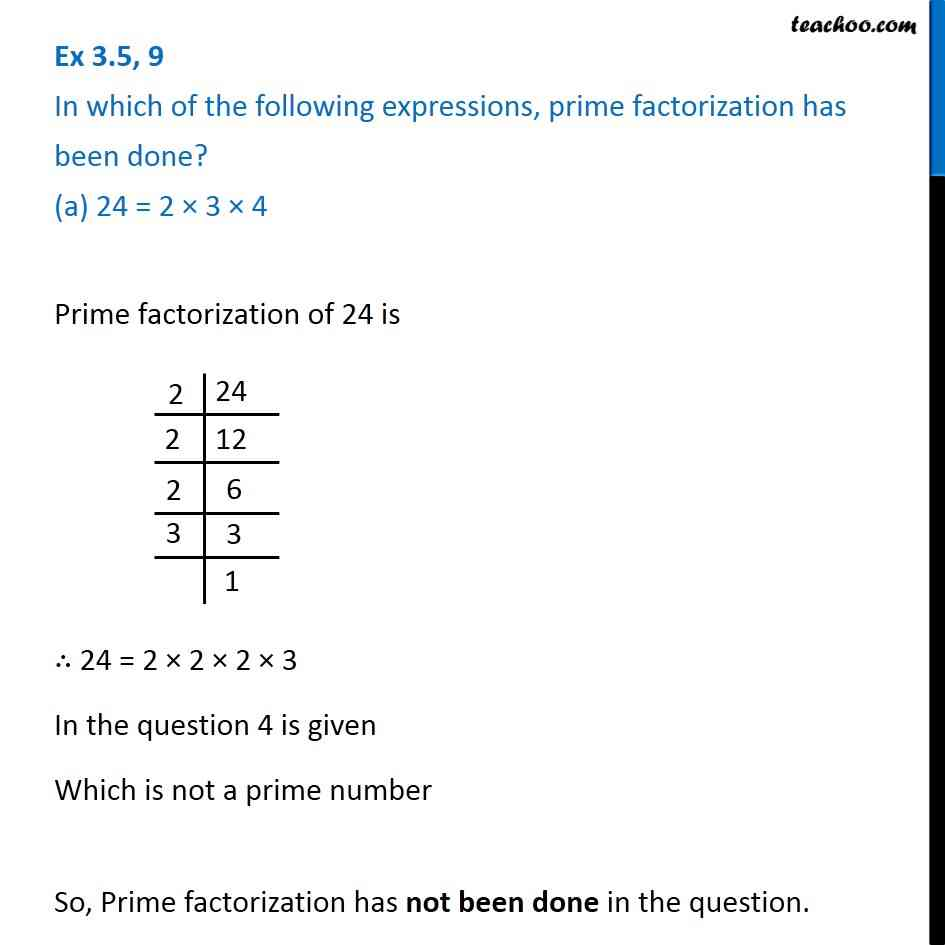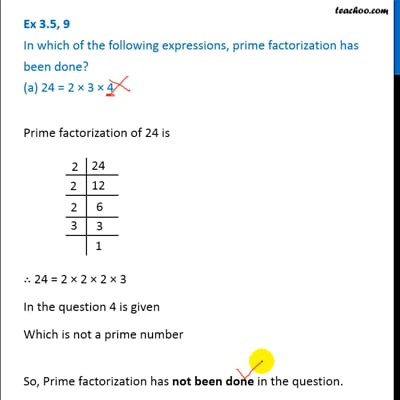This video is only available for Teachoo black users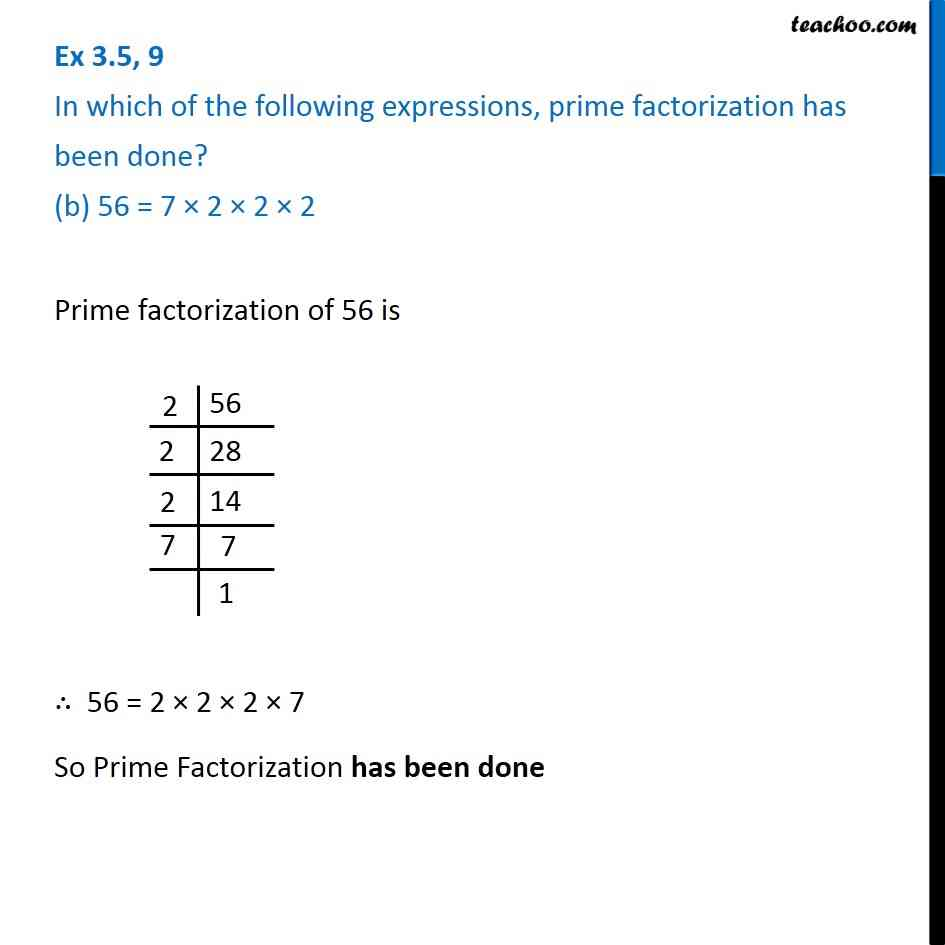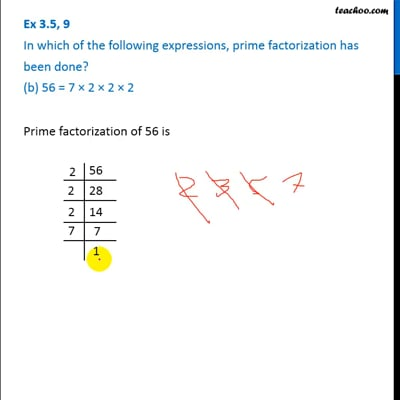This video is only available for Teachoo black users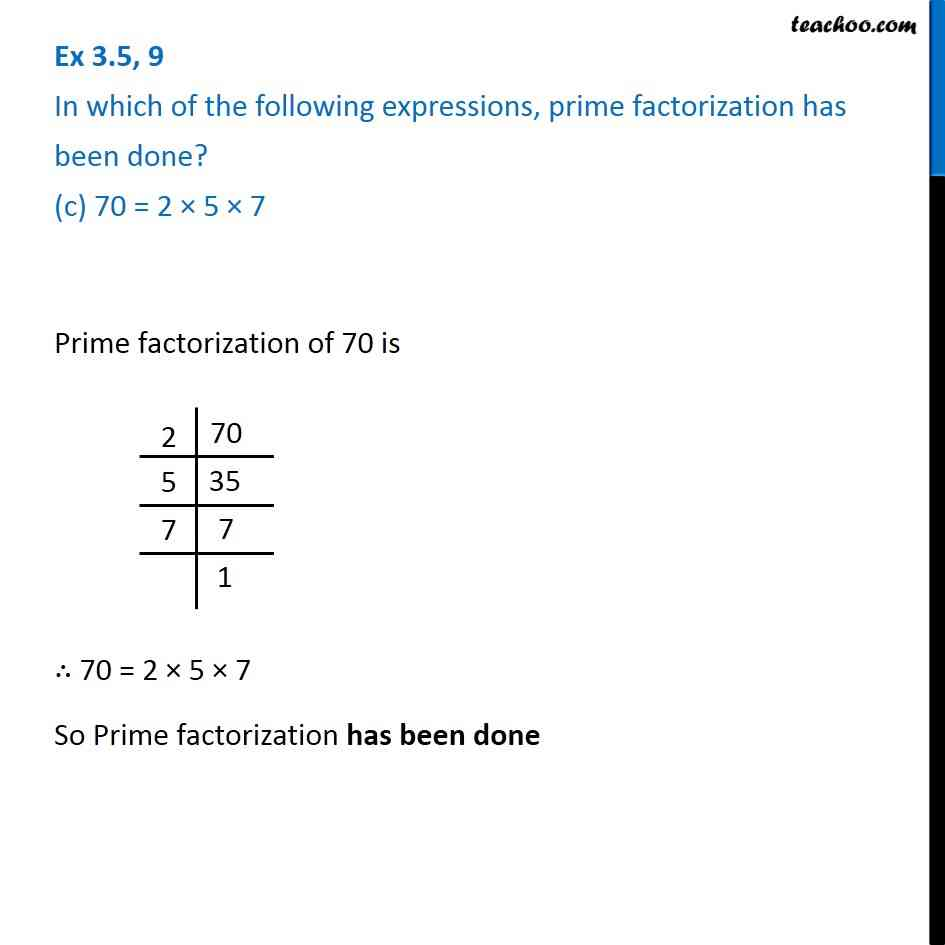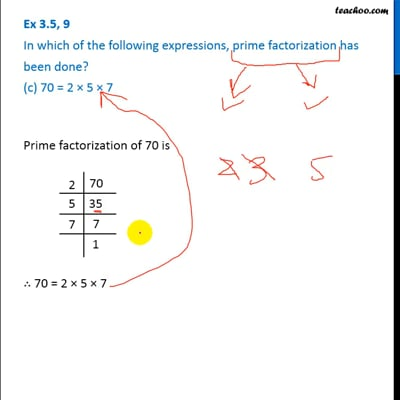This video is only available for Teachoo black users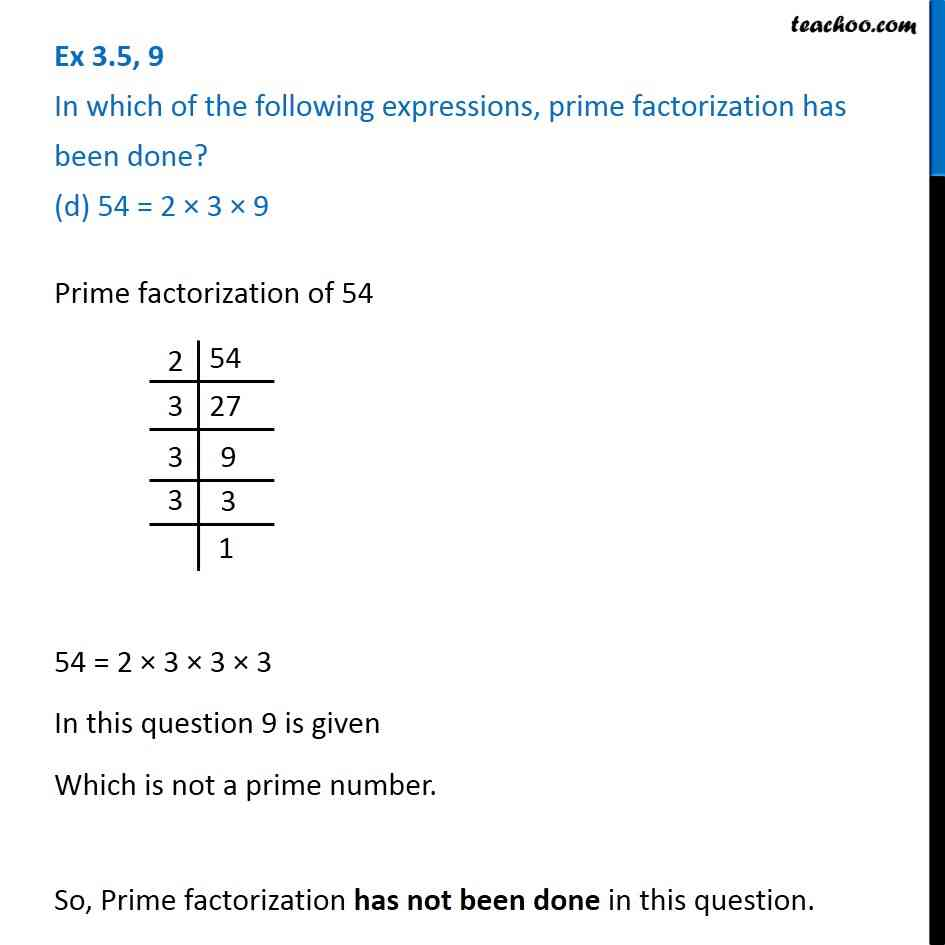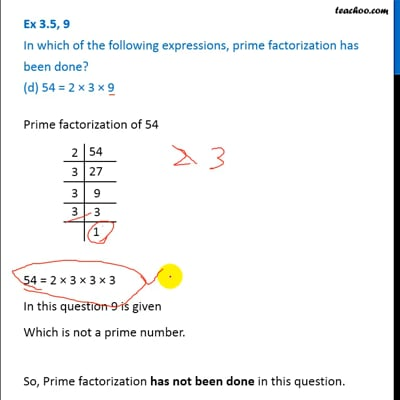This video is only available for Teachoo black users

Solve all your doubts with Teachoo Black (new monthly pack available now!)

### Transcript

Ex 3.5, 9 In which of the following expressions, prime factorization has been done? (a) 24 = 2 × 3 × 4Prime factorization of 24 is ∴ 24 = 2 × 2 × 2 × 3 In the question 4 is given Which is not a prime number So, Prime factorization has not been done in the question. Ex 3.5, 9 In which of the following expressions, prime factorization has been done? (b) 56 = 7 × 2 × 2 × 2Prime factorization of 56 is ∴ 56 = 2 × 2 × 2 × 7 So Prime Factorization has been done Ex 3.5, 9 In which of the following expressions, prime factorization has been done? (c) 70 = 2 × 5 × 7 Prime factorization of 70 is ∴ 70 = 2 × 5 × 7 So Prime factorization has been done Ex 3.5, 9 In which of the following expressions, prime factorization has been done? (d) 54 = 2 × 3 × 9Prime factorization of 54 54 = 2 × 3 × 3 × 3 In this question 9 is given Which is not a prime number. So, Prime factorization has not been done in this question.